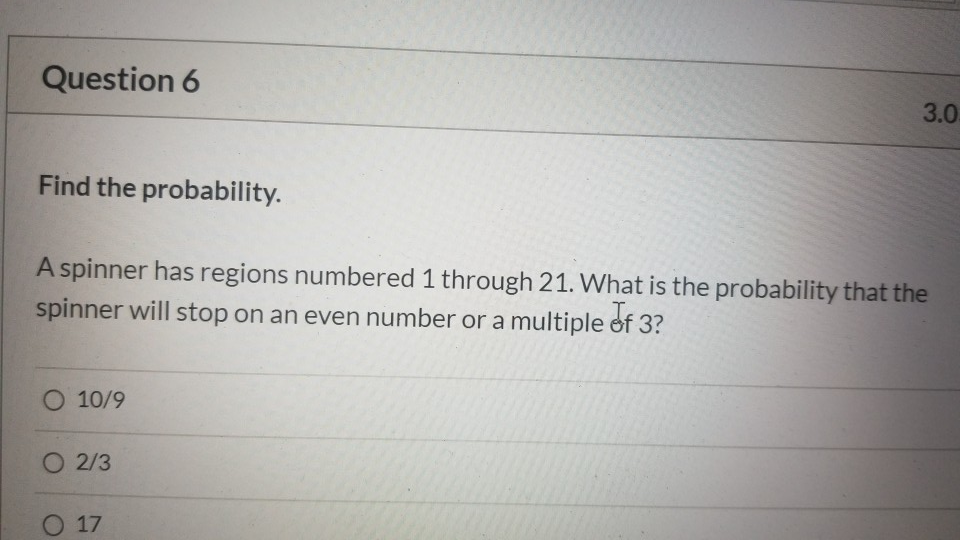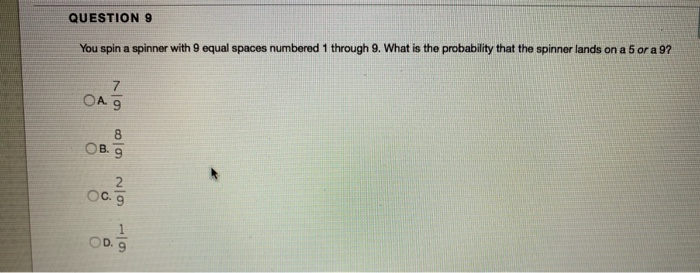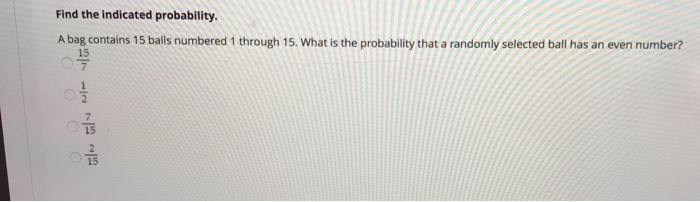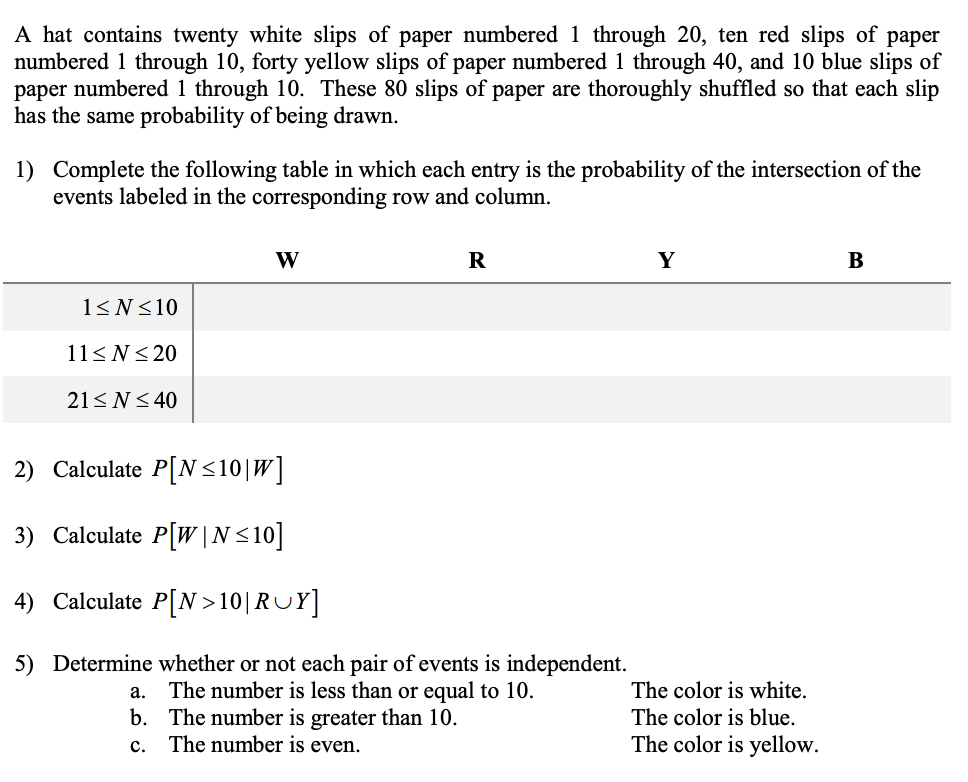Question

a spinner has regions numbered through 1-21 what is the probability that the spinner will stop on an even number or a multiple of 3

a spinner has regions numbered through 1-21

what is the probability that the spinner will stop on an even number or a multiple of 3..

I am confused.
I keep coming up with 17/21

but the real answer is 2/3

how how how ?

Even number = 10/21

Multiple of 3 = 7/21

10/21 + 7/21 = 17/21

Guess what? I keep coming up with 17/21 too.

Book typo?

you must subtract 3/21= 14/21. then simplify, which gives you 2/3.

Earn Coins

Coins can be redeemed for fabulous gifts.

Similar Homework Help Questions
• A spinner has regions numbered 1 through 21

A spinner has regions numbered 1 through 21. What is the probability that the spinner will stop on an even number or a multiple of 3?Okay so I am lost. I understand that the probability of an event is the # of ways an event can occur over the total # of possible outcomes.I need some explaining done. Please

• Question 6 3.0 Find the probability. A spinner has regions numbered 1 through 21. What is...Question 6 3.0 Find the probability. A spinner has regions numbered 1 through 21. What is the probability that the spinner will stop on an even number or a multiple of 3? O 10/9 O 2/3 O 17

• A spinner has ten regions numbered 1 through 10

A spinner has ten regions numbered 1 through 10. if the spinner is spun 100 times we would expect about 10 of the outcomes to be region 1. it can be determined that the spinner is unbalanced if x the number of outcomes in region 1 satisfies: {(x-10)/3} 1.645 Describe the number of outcomes that determine an unbalanced spinner that is spun 100 times.

• if a spinner is numbered 1-10 what is the probability of it stopping on a multiple of 3

if a spinner is numbered 1-10 what is the probability of it stopping on a multiple of 3?

• A lottery game has balls numbered from 0 - 9 If a ball is selected at random, what is the probability of selecting an even numbered ball or a 5

A lottery game has balls numbered from 0 - 9 If a ball is selected at random, what is the probability of selecting an even numbered ball or a 5 ?I am guessing this is Mutually exclusive event and hence used the OR= P(A)+P(B) conceptconsidering 0 as an even number I keep getting 1 as an answer..help me out.. am I doing it wrong?

• A spinner is divided into 5 equal sections numbered 1, 2, 3, 4, and 5

A spinner is divided into 5 equal sections numbered 1, 2, 3, 4, and 5. If the spinner is spun and a coin is tossed, what is the probability of getting a tail on the coin and an odd number on the spinner

• QUESTION 9 You spin a spinner with 9 equal spaces numbered 1 through 9. What is...QUESTION 9 You spin a spinner with 9 equal spaces numbered 1 through 9. What is the probability that the spinner lands on a 5 or a 9? 7 ОА 9 8 ов. 9 2 OC. 9 1 OD 9

• A lottery game has balls numbered 1 through 15

A lottery game has balls numbered 1 through 15. If a ball is selected at random, what is the probability of selecting a ball showing an even number or the number 12?I thought the answer would be 7 even numbers and 1 for the 12 which would be 8/15 is not an option for the answer.Our choices are 5/4,7,4/5,7/15

• Find the indicated probability. A bag contains 15 balls numbered 1 through 15. What is the...Find the indicated probability. A bag contains 15 balls numbered 1 through 15. What is the probability that a randomly selected ball has an even number? 15

• A hat contains twenty white slips of paper numbered 1 through 20, ten red slips of...A hat contains twenty white slips of paper numbered 1 through 20, ten red slips of paper numbered 1 through 10, forty yellow slips of paper numbered 1 through 40, and 10 blue slips of paper numbered 1 through 10. These 80 slips of paper are thoroughly shuffled so that each slip has the same probability of being drawn 1) Complete the following table in which each entry is the probability of the intersection of the events labeled in the...